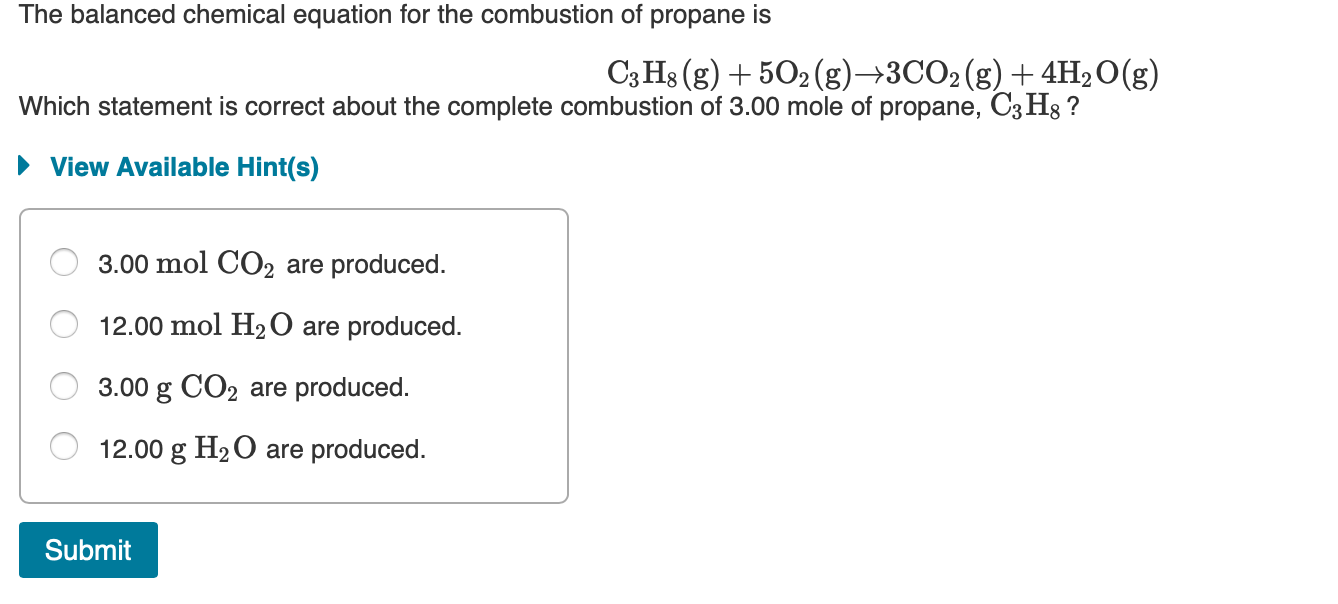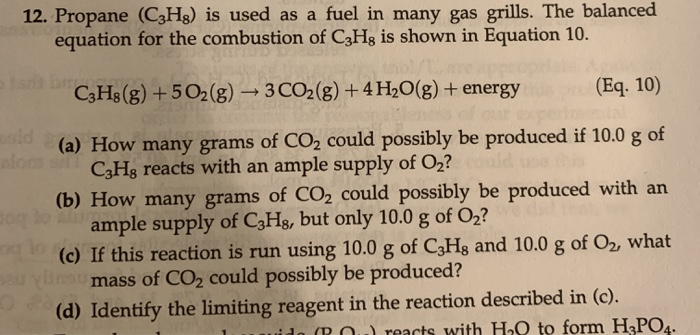Question

# Many home barbeques are fueled with propane gas C3H8

Many home barbeques are fueled with propane gas C3H8.

What mass of carbon dioxide (in kg) is produced upon the complete combustion of 18.9L  of propane (approximate contents of one 5-gallon tank)? Assume that the density of the liquid propane in the tank is 0.621g/ml.

How do I start & work this question?

I wrote a balanced equation:
C3H8 + 5O2 -> 4H2O + 3CO2

Now, where do I go from here?

The propane is liquid. Determine the mass from the volume and liquid. Convert that mass to moles.

For each mole of propane, you get (from the balanced equation) three times as much CO2. Convert moles of carbon dioxide to kg

#### Earn Coins

Coins can be redeemed for fabulous gifts.

Similar Homework Help Questions
• ### I am still not quite grasping how to complete this problem: Posted by K on Sunday, October 14, 2007 at 6:43pm

I am still not quite grasping how to complete this problem:Posted by K on Sunday, October 14, 2007 at 6:43pm. Many home barbeques are fueled with propane gas C3H8. What mass of carbon dioxide (in kg) is produced upon the complete combustion of 18.9L of propane (approximate contents of one 5-gallon tank)? Assume that the density of the liquid propane in the tank is 0.621g/ml. How do I start & work this question? I wrote a balanced equation: C3H8 +...

• ### Many home barbecues are fueled with propane gas (C3H8)

Many home barbecues are fueled with propane gas (C3H8). How much carbon dioxide in kilograms is produced upon the complete combustion of 18.9 L of propane (approximate contents of one 5-gal tank)? Assume that the density of the liquid propane in the tank is 0.621g/mL.

• ### The propane fuel (C3H8) used in gas barbeques burns according to the following thermochemical equation: C3H8(g)+5O2(g)...

The propane fuel (C3H8) used in gas barbeques burns according to the following thermochemical equation: C3H8(g)+5O2(g)?3CO2(g)+4H2O(g) If a pork roast must absorb 1700kJ to fully cook, and if only 14% of the heat produced by the barbeque is actually absorbed by the roast, what mass of CO2 is emitted into the atmosphere during the grilling of the pork roast? Express your answer using two significant figures.

• ### The propane fuel (C3H8) used in gas barbeques burns according to the following thermochemical equation: C3H8(g)+5O2(g)...

The propane fuel (C3H8) used in gas barbeques burns according to the following thermochemical equation:C3H8(g)+5O2(g)→3CO2(g)+4H2O(g) ΔH rxn= 2217kJPart AIf a pork roast must absorb 1500kJ to fully cook, and if only 12% of the heat produced by the barbeque is actually absorbed by the roast, what mass of CO2 is emitted into the atmosphere during the grilling of the pork roast?Express your answer using two significant figures. m=_____________________g

• ### The propane fuel (C3H8) used in gas barbeques burns according to this thermochemical equation. C3H8(g)+5O2(g)→3CO2(g)+4H2O(g)ΔH∘rxn=−2044kJ If...

The propane fuel (C3H8) used in gas barbeques burns according to this thermochemical equation. C3H8(g)+5O2(g)→3CO2(g)+4H2O(g)ΔH∘rxn=−2044kJ If a pork roast must absorb 1.7×103 kJ to fully cook, and if only 15 % of the heat produced by the barbeque is actually absorbed by the roast, what mass of CO2 is emitted into the atmosphere during the grilling of the pork roast?

• ### If you start with #7.0# moles of propane and #7.0# moles of oxygen gas what is the percent yield if #4.0# moles of carbon dioxide are produced?

Consider the reaction below. If you start with 7.0 moles of C3H8 (propane) and 7.0 moles of O2, _ is the percent yield if 4.0 moles of carbon dioxide is produced. C3H8(g) + 5O2(g) --> 3CO2(g) + 4H2O(g)

• ### The balanced chemical equation for the combustion of propane is C3H8(g) + 5O2(g) +3CO2(g) + 4H2O(g)...The balanced chemical equation for the combustion of propane is C3H8(g) + 5O2(g) +3CO2(g) + 4H2O(g) Which statement is correct about the complete combustion of 3.00 mole of propane, C3Hg ? ► View Available Hint(s) O 3.00 mol CO2 are produced. O 12.00 mol H2O are produced. O 3.00 g CO2 are produced. O 12.00 g H2O are produced. Submit

• ### Liquid propane (C3H8) is a common heating fuel in rural Nevada where there is no access...

Liquid propane (C3H8) is a common heating fuel in rural Nevada where there is no access to a natural gas pipeline. An average home will use roughly 1000 gallons of liquid propane for heating during a year if this is the only source of heating. Given that a gallon is 3.79 liters and the density of liquid propane is 0.493g/cm3, how many grams of carbon dioxide are produced in the combustion of 1000 gallons of propane needed to heat a...

• ### 12. Propane (C3H8) is used as a fuel in many gas grills. The balanced equation for the combustion of C3Hg is shown...12. Propane (C3H8) is used as a fuel in many gas grills. The balanced equation for the combustion of C3Hg is shown in Equation 10. C3H8(g) +502(g) + 3 CO2(g) + 4H2O(g) + energy (Eq. 10) (a) How many grams of CO2 could possibly be produced if 10.0 g of CzHg reacts with an ample supply of Oz? (b) How many grams of CO2 could possibly be produced with an ample supply of C3Hg, but only 10.0 g of O2?...

• ### A fuel gas consists of 75% butane (C4H10), 10% propane (C3H8) and 15% butene (C4H8) by...

A fuel gas consists of 75% butane (C4H10), 10% propane (C3H8) and 15% butene (C4H8) by volume. It is to be fed to the combustion chamber in 10% excess air at 25ºC, where it is completely burnt to carbon dioxide and water. The flue gases produced are to be used to generate 5 bar steam from water at 90ºC. net calorific value (MJm-3) at 25oC Butane (C4H10) = 111.7MJm-3, Butene (C4H8) = 105.2 MJm-3. Propane (C3H8) = 85.8 MJm-3. air...